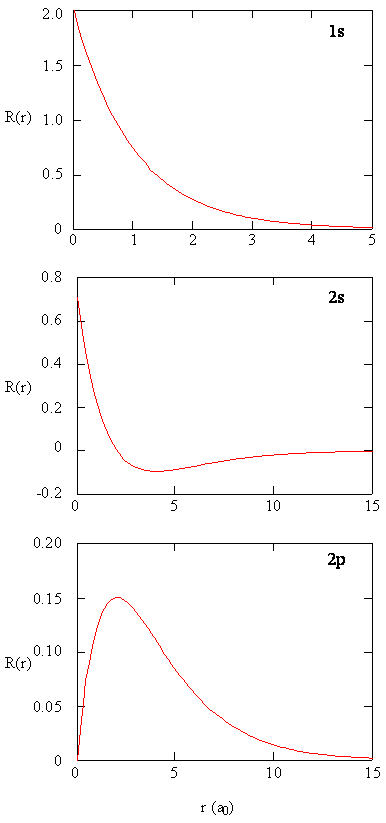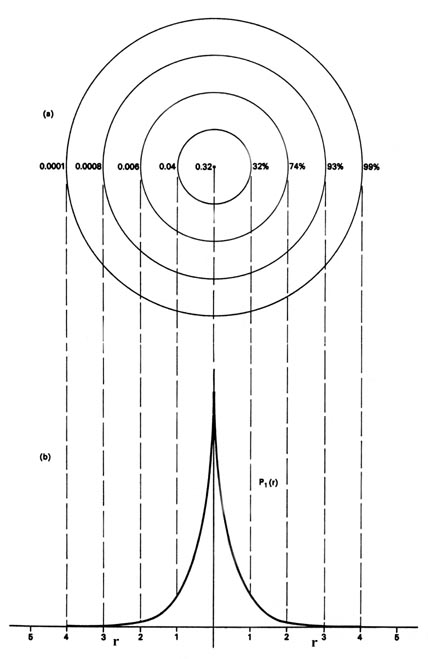17: Hydrogen-like Solutions

•• Contributed by Delmar Larsen
• Founder and Director at Libretexts

Recap of Lecture 15

Last lecture we completed the discussion of Rigid Rotors within the context of microwave spectroscopy (a topic of Worksheet 4B: Rotational Spectroscopy). We introduce the hydrogen atom (the most important model and real system for quantum chemistry), by defining the potential, Hamiltonian and Schrodinger equation. We argued the solution of the Schodinger equation involves a radial component and an angular component. The latter is just the spherical harmonics derived in the rigid rotor system previously. We ended lecture on the radial component which is a function of four terms: A normalization constant, associated Laguerre polynomial, a nodal function, and an exponential decay. We also discussed that the energy is a function of only one quantum number and that there is a degeneracy of the wavefunctions.

Java simulation of particles in boxes :https://phet.colorado.edu/en/simulation/bound-states

Recap of Energy

The motion of the electron in the hydrogen atom is not free. The electron is bound to the atom by the attractive force of the nucleus and consequently quantum mechanics predicts that the total energy of the electron is quantized. The expression for the energy is:

$E_n =\dfrac{-2 \pi^2 m e^4Z^2}{n^2h^2} \label{17.1}$

with $$n = 1,2,3,4...$$

where $$m$$ is the mass of the electron, $$e$$ is the magnitude of the electronic charge, $$n$$ is a quantum number, $$h$$ is Planck's constant and $$Z$$ is the atomic number (the number of positive charges in the nucleus). Equation $$\ref{17.1}$$ applies to any one-electron atom or ion. For example, He+ is a one-electron system for which Z = 2. We can again construct an energy level diagram listing the allowed energy values (Figure $$\PageIndex{1}$$).Figure $$\PageIndex{1}$$: The energy level diagram for the H atom. Each line denotes an allowed energy for the atom. An energy-level diagram plots energy vertically and is useful in visualizing the energy states of a system and the transitions between them. This diagram is for the hydrogen-atom electrons.

These are obtained by substituting all possible values of n into Equation $$\ref{17.1}$$. As in our previous example, we shall represent all the constants which appear in the expression for $$E_n$$ by teh Rydberg constant $$R$$ and we shall set $$Z = 1$$, i.e., consider only the hydrogen atom.

$E_n = \dfrac{-R}{n^2} \label{17.2}$

with $$n = 1,2,3,4...$$.

Example $$\PageIndex{1}$$:

How much energy (in eV) is required to remove an electron from each of the following orbitals in a hydrogen atom:

1. 2p
2. 4d

The removal of an electron from a Hydrogen atom is akin to moving an electron from energy state n to a distance infinity. The energy required for this is called the ionization energy which is equivalent (with the possible exception of a sign change depending on how ionization energy is defined) to the energy of a one-electron atom like the Hydrogen atom. Thus, solve for the desired energy using the following equation for the energy of a one-electron atom where Eh is the Hartree energy:

$E_n = \dfrac{Z^2 E_h}{2 n^2}$

1. $$E_2 = \dfrac{(1)^2 (27.211\;eV)}{2 (2)^2} = 3.40\;eV$$
2. $$E_4 = \dfrac{(1)^2 (27.211\;eV)}{2 (3)^2} = 0.850\;eV$$

Radial Part of the Wavefunction (ignoring angular part)

The Spherical Harmonic functions provide information about where the electron is around the proton, and the radial function R(r) describes how far the electron is away from the proton. The $$R(r)$$ functions that solve the radial differential Equation $$\ref{6.1.14}$$, are products of the associated Laguerre polynomials times the exponential factor, multiplied by a normalization factor $$(N_{n,l})$$ and $$\left (\dfrac {r}{a_0} \right)^l$$.

$\color{red} R (r) = N_{n,l} \left ( \dfrac {r}{a_0} \right ) ^l L_{n,l} (r) e^{-\frac {r}{n {a_0}}} \label{6.1.17}$

The decreasing exponential term overpowers the increasing polynomial term so that the overall wavefunction exhibits the desired approach to zero at large values of $$r$$. The first six radial functions are provided below. Note that the functions in the table exhibit a dependence on $$Z$$, the atomic number of the nucleus. As discussed later in this chapter, other one electron systems have electronic states analogous to those for the hydrogen atom, and inclusion of the charge on the nucleus allows the same wavefunctions to be used for all one-electron systems. For hydrogen, $$Z = 1$$.

n
$$l$$
$$R_{n,l} (r)$$
Radial functions for one-electron atoms and ions. $$Z$$ is the atomic number of the nucleus, and $$\rho = \dfrac {Zr}{a_0}$$, where $$a_0$$ is the Bohr radius and r is the radial variable.
1
0
$$2 \left (\dfrac {Z}{a_0} \right )^{3/2} e^{-\rho}$$
2
0
$$\dfrac {1}{2 \sqrt {2}}\left (\dfrac {Z}{a_0} \right )^{3/2} (2 - \rho) e^{-\rho/2}$$
2
1
$$\dfrac {1}{2 \sqrt {6}}\left (\dfrac {Z}{a_0} \right )^{3/2} \rho e^{-\rho/2}$$
3
0
$$\dfrac {1}{81 \sqrt {3}}\left (\dfrac {Z}{a_0} \right )^{3/2} (27 - 18 \rho + 2\rho ^2) e^{-\rho/3}$$
3
1
$$\dfrac {1}{81 \sqrt {6}}\left (\dfrac {Z}{a_0} \right) ^{3/2} (6 \rho + \rho ^2) e^{-\rho/3}$$
3
2
$$\dfrac {1}{81 \sqrt {30}}\left (\dfrac {Z}{a_0} \right ) ^{3/2} \rho ^2 e^{-\rho/3}$$

The constraint that $$n$$ be greater than or equal to $$l +1$$ also turns out to quantize the energy, producing the same quantized expression for hydrogen atom energy levels that was obtained from the Bohr model of the hydrogen atom.

$E_n = - \dfrac {m_e e^4}{8 \epsilon ^2_0 h^2 n^2}$

Comparison to Bohr Theory

It is interesting to compare the results obtained by solving the Schrödinger equation with Bohr’s model of the hydrogen atom. There are several ways in which the Schrödinger model and Bohr model differ.

1. First, and perhaps most strikingly, the Schrödinger model does not produce well-defined orbits for the electron. The wavefunctions only give us the probability for the electron to be at various directions and distances from the proton.
2. Second, the quantization of angular momentum is different from that proposed by Bohr. Bohr proposed that the angular momentum is quantized in integer units of $$\hbar$$, while the Schrödinger model leads to an angular momentum of $$\sqrt{(l (l +1) \hbar ^2}$$.
3. Third, the quantum numbers appear naturally during solution of the Schrödinger equation while Bohr had to postulate the existence of quantized energy states. Although more complex, the Schrödinger model leads to a better correspondence between theory and experiment over a range of applications that was not possible for the Bohr model.

Methods for separately examining the radial portions of atomic orbitals provide useful information about the distribution of charge density within the orbitals.Figure 6.2.2: Radial function, R(r), for the 1s, 2s, and 2p orbitals.

We could also represent the distribution of negative charge in the hydrogen atom in the manner used previously for the electron confined to move on a plane (Figure $$\PageIndex{1}$$), by displaying the charge density in a plane by means of a contour map. Imagine a plane through the atom including the nucleus. The density is calculated at every point in this plane. All points having the same value for the electron density in this plane are joined by a contour line (Figure $$\PageIndex{3}$$). Since the electron density depends only on r, the distance from the nucleus, and not on the direction in space, the contours will be circular. A contour map is useful as it indicates the "shape" of the density distribution.Figure $$\PageIndex{1}$$ for $$P_1$$, but now plotted from the nucleus in both directions.
$$n$$

$$\ell$$

$$m$$ Eigenstates
Hydrogen-like atomic wavefunctions for $$n$$ values $$1,2,3$$: $$Z$$ is the atomic number of the nucleus, and $$\rho = \dfrac {Zr}{a_0}$$, where $$a_0$$ is the Bohr radius and r is the radial variable.
$$n=1$$ $$\ell=0$$ $$m=0$$ $$\psi_{100} = \dfrac {1}{\sqrt {\pi}} \left(\dfrac {Z}{a_0}\right)^{\frac {3}{2}} e^{-\rho}$$
$$n=2$$ $$\ell=0$$ $$m=0$$ $$\psi_{200} = \dfrac {1}{\sqrt {32\pi}} \left(\dfrac {Z}{a_0}\right)^{\frac {3}{2}} (2-\rho)e^{\dfrac {-\rho}{2}}$$
$$\ell=1$$ $$m=0$$ $$\psi_{210} = \dfrac {1}{\sqrt {32\pi}} \left(\dfrac {Z}{a_0}\right)^{\frac {3}{2}} \rho e^{-\rho/2} \cos(\theta)$$
$$\ell=1$$ $$m=\pm 1$$ $$\psi_{21\pm\ 1} = \dfrac {1}{\sqrt {64\pi}} \left(\dfrac {Z}{a_0}\right)^{\dfrac {3}{2}} \rho e^{-\rho/2} \sin(\theta) e^{\pm\ i\phi}$$
$$n=3$$ $$\ell=0$$ $$m=0$$ $$\psi_{300} = \dfrac {1}{81\sqrt {3\pi}} \left(\dfrac {Z}{a_0}\right)^{\frac {3}{2}} (27-18\rho +2\rho^2)e^{-\rho/3}$$
$$\ell=1$$ $$m=0$$ $$\psi_{310} = \dfrac{1}{81} \sqrt {\dfrac {2}{\pi}} \left(\dfrac {Z}{a_0}\right)^{\dfrac {3}{2}} (6r - \rho^2)e^{-\rho/3} \cos(\theta)$$
$$\ell=1$$ $$m=\pm 1$$ $$\psi_{31\pm\ 1} = \dfrac {1}{81\sqrt {\pi}} \left(\dfrac {Z}{a_0}\right)^{\frac {3}{2}} (6\rho - \rho^2)e^{-r/3} \sin(\theta)e^{\pm\ i \phi}$$
$$\ell=2$$ $$m=0$$ $$\psi_{320} = \dfrac {1}{81\sqrt {6\pi}} \left(\dfrac {Z}{a_0}\right)^{\frac {3}{2}} \rho^2 e^{-\rho/3}(3cos^2(\theta) -1)$$
$$\ell=2$$ $$m=\pm 1$$ $$\psi_{32\pm\ 1} = \dfrac {1}{81\sqrt {\pi}} \left(\dfrac {Z}{a_0}\right)^{\frac {3}{2}} \rho^2 e^{-\rho/3} \sin(\theta)\cos(\theta)e^{\pm\ i \phi}$$
$$\ell=2$$ $$m=\pm 2$$

$$\psi_{32\pm\ 2} = \dfrac {1}{162\sqrt {\pi}} \left(\dfrac {Z}{a_0}\right)^{\frac {3}{2}} \rho^2 e^{-\rho/3}{\sin}^2(\theta)e^{\pm\ 2i\phi}$$

Volume and Surface Elements in Spherical Coordinates

In Cartesian space, the volume element that one integrates over to extract a probability is

$dV = dx\,dy\,dz$

while there are straightforward approaches to convert $$(x,y,z)$$ to $$r,\phi,\theta$$, the corresponding volume element requires the Jacobian to be introduced (as expected for all transformations).So the volume element spanning from $$r$$ to $$r+dr$$ $$\theta$$ to $$\theta + d\theta$$, and $$\phi$$ to $$\phi + d\phi$$ is

$\mathrm{d}V=r^2 \sin \theta \,\mathrm{d}r\,\mathrm{d}\theta\,\mathrm{d}\varphi.$

The surface element spanning from$$\theta + d\theta$$, and $$\phi$$ to $$\phi + d\phi$$ on a spherical surface at (constant) radius $$r$$ is

$\mathrm{d}S_r=r^2\sin\theta\,\mathrm{d}\theta\,\mathrm{d}\varphi.$Surface area element dA and volume dV of a thin spherical shell, containing all the light sources at coordinate distance r.

The quantity $$R(r)^* R(r)$$ gives the radial probability density; i.e., the probability density for the electron to be at a point located the distance r from the proton. Radial probability densities for three types of atomic orbitals are plotted below.Radial probability densities for the 1s, 2s, and 2p orbitals.

When the radial probability density for every value of $$r$$ is multiplied by the area of the spherical surface element ($$dS$$ represented by that particular value of $$r$$, we get the radial distribution function. The radial distribution function gives the probability density for an electron to be found anywhere on the surface of a sphere located a distance $$r$$ from the proton. Since the area of a spherical surface is $$4 \pi r^2$$, the radial distribution function is given by

$\color{red} 4 \pi r^2 R(r)^* R(r)$Figure $$\PageIndex{5}$$: Radial distribution functions for the 1s, 2s, and 2p density distributions. Notice the number of nodes in each distribution.

Example: Most probable radius

Show that for a 1s orbital of a hydrogen-like atom the most probable distance from proton to electron is a0/Z.

Solution

$\psi_{1s} = \psi_{1,0,0}(r,\theta,\phi) = R_{1,0}(r)Y_{0,0}(\theta,\phi) = \dfrac{1}{\sqrt{\pi}}(\dfrac{Z}{a_0})^{\dfrac{3}{2}}exp(-Zr/a_0)$

Where Z is the atomic number and a0 is the Bohr radius(5.29x10-11m).

The radial probability density for this state is:

$\langle \psi_{1,0,0}|r^2|\psi_{1,0,0} \rangle= \dfrac{1}{\pi}(\dfrac{Z}{a_0})^3r^2exp(-Zr/a_0)$

To find the most probable distance from proton to electron,

$\dfrac{d}{dr}\langle \psi_{1,0,0}|r^2|\psi_{1,0,0} \rangle = \dfrac{d}{dr}(\dfrac{1}{\pi}(\dfrac{Z}{a_0})^3r^2exp(-Zr/a_0)$

$\dfrac{d}{dr}\langle \psi_{1,0,0}|r^2|\psi_{1,0,0} \rangle = \dfrac{1}{\pi}(\dfrac{Z}{a_0})^3(2r-\dfrac{2Z}{a_0}exp(-Zr/a_0)$

$\dfrac{1}{\pi}(\dfrac{Z}{a_0})^3(2r-\dfrac{2Z}{a_0})exp(-Zr/a_0) = 0$

$2r-\dfrac{2Z}{a_0} = 0$

$r = \dfrac{a_0}{Z}$

Example: Probability

Calculate the probability of finding a 1s hydrogen electron being found within distance $$2a_o$$ from the nucleus.

Solution

Note the wavefunction of hydrogen 1s orbital which is

$ψ_{100}= \dfrac{1}{\sqrt{π}} \left(\dfrac{1}{a_0}\right)^{3/2} e^{-\rho}$

with $$\rho=\dfrac{r}{a_0}$$.

The probability of finding the electron within $$2a_0$$ distance from the nucleus will be:

$prob= \underbrace{\int_{0}^{\pi} \sin \theta \, d\theta}_{over\, \theta} \, \overbrace{ \dfrac{1}{\pi a_0^3} \int_{0}^{2a_0} r^2 e^{-2r/a_0} dr}^{over\, r} \, \underbrace{ \int_{0}^{2\pi} d\phi }_{over\, \phi }$

Since $$\int_0^{\pi} \sin \theta d\theta=2$$ and $$\int_0^{2\pi} d\phi=2\pi$$, we have

$prob=2 \times 2\pi \times \dfrac{1}{\pi a_0^3} \int_0^2a_0 (-a_0/2)r^2 d e^{-2r/a_0}$

$=\dfrac{4}{a_0^3}(-\dfrac{a_0}{2}) (r^2 e^{-2r/a_0} |_0^{2a_0} - \int_0^{2a_0} 2r e^{-2r/a_0} dr)$

$= -\dfrac{2}{a_0^2} [(2a_0)^2 e^{-4}-0-2\int_0^{2a_0} r (-\dfrac{a_0}{2}) d e^{-2r/a_0} ]$

$=-\dfrac{2}{a_0^2}4a_0^2 e^{-4} +\dfrac{4}{a_0^2}(-\dfrac{a_0}{2}) (r e^{-2r/a_0} |_0^{2a_0}-\int_0^{2a_0} e^{-2r/a_0} dr )$

$=-8e^{-4}-\dfrac{2}{a_0} \left[2a_0e^{-4}-0-(-\dfrac{a_0}{2})e^{-2r/a_0} |_0^{2a_0} \right]$

$=-8e^{-4}-4e^{-4}-e^{2r/a_0} |_0^{2a_0}$

$=-12 e^{-4}-(e^{-4}-1)=1-13e^{-4}=0.762$

There is a 76.2% probability that the electrons will be within $$2a_o$$ of the nucleus in the 1s eigenstate.